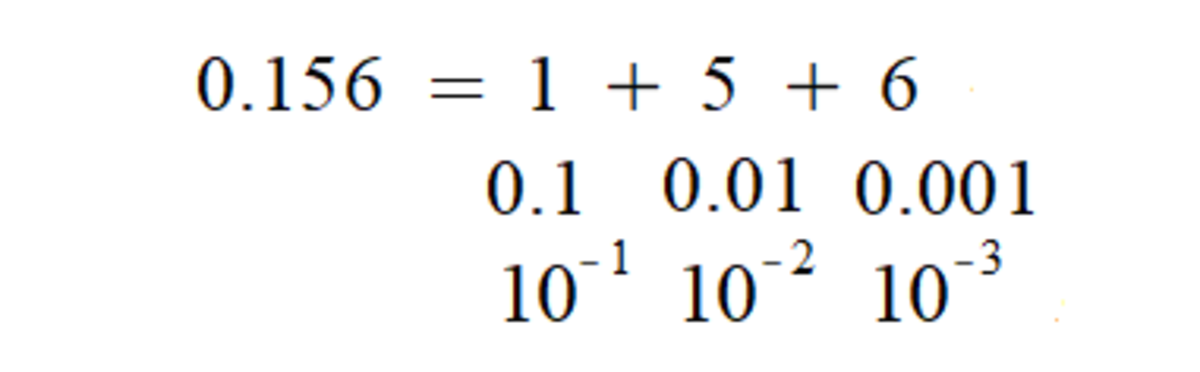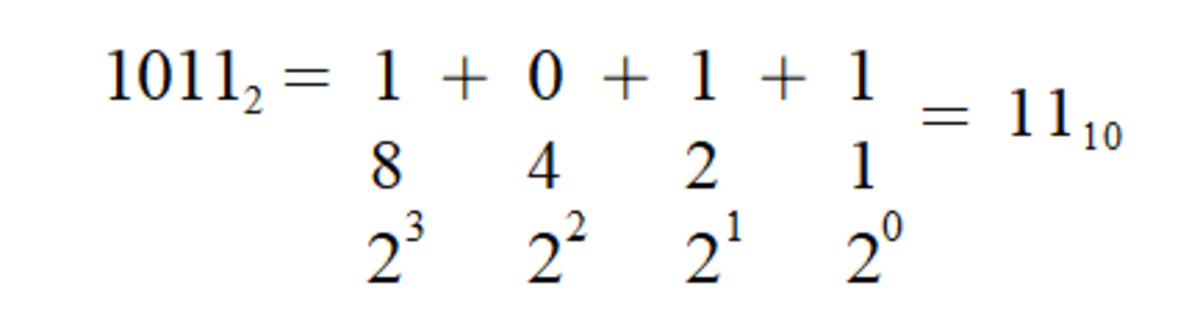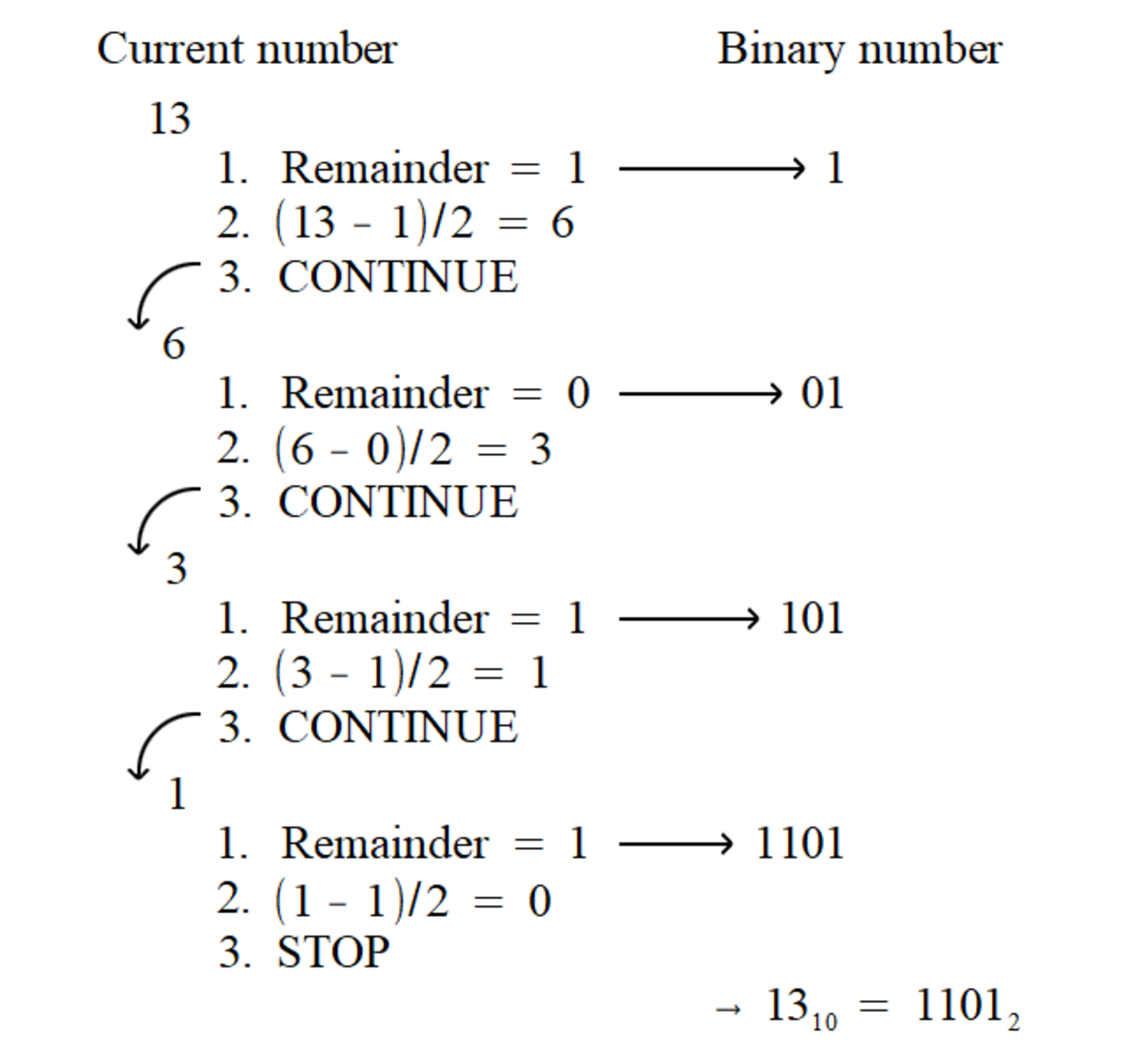# Alternative Number Systems: What Are Binary Numbers?

Sam Brind holds a master's in physics with theoretical physics (MPhys) from the University of Manchester.

## What is a number system?

Number systems define how numbers are represented when written down. Numbers are written down as a collection of symbols, known as digits. Each digit is used to signify a numeric contribution towards the value of the total number. Modern numbers systems are positional and defined around a base number (less commonly called the radix). A positional system means that the contribution depends on the digit's position within the number's collection of digits. Specifically, each digit represents a multiple of the base number raised to a specific power; the further leftward the digit is placed, the greater the power. The base number defines the range of possible values that a digit can take.

The number system used within everyday life is called the decimal number system and is based on the number ten. The choice of ten probably correlates with its convenience for counting in the earliest use of numbers. It also matches the fact that we each have ten fingers (also referred to as digits).

Computers store numbers as binary data. When discussing computer calculations, it is, therefore, essential to represent numbers in the binary number system, which uses two as the base. The hexadecimal number system, which uses sixteen as the base, is another commonly used number system for analysing computer data. Hexadecimal allows binary numbers to be represented in a more concise and readable manner.

## Decimal (Base-10)

The range of digits allowed by decimal (also referred to as denary) includes 0, 1, 2, 3, 4, 5, 6, 7, 8, and 9. This follows from a more general principle, which dictates that the allowed set of digits for a base-N system are the numbers from 0 through to N-1.

The example below demonstrates how the digits of the number 3265 represent contributions that sum towards the number: three lots of 1000 plus two lots of 100 plus 6 lots of 10 and 5 lots of 1.A breakdown of what the denary representation of 3265 actually means. Each digit corresponds to a power of ten (increasing from right to left). The number is then given by summing these contributions together.

Any digits placed after the decimal point follow the pattern of the power of ten decreasing. Negative powers of ten allow fractional numbers to be represented.A breakdown of what the denary representation of 0.156 actually means.

## Binary (Base-2)

Binary numbers have only two digits: either 0 or 1. The smallest piece of data stored by a computer is called a bit, short for binary digit. Computers are built to store data in bits because they only require two distinct states; this is simple to build and allows data to be robust to interference from electrical noise.A breakdown of the binary representation of eleven. Notice the pattern is the same as previously shown for decimal numbers but with the base switched to two. The base used in representing a number can be indicated through the use of a subscript.

While bits are the fundamental pieces of computer data, it's more common to think about data in terms of bytes. One byte is made up of eight bits. Hexadecimal is commonly used because it allows a byte to be represented by just two digits. This allows long binary numbers to be reduced to a much more compact form.

Hexadecimal allows digits that are ten or larger, which has the potential to be very confusing when written down. Typically, the characters A-F are used as a substitute for the digits ten to fifteen. Therefore, the range of possible hexadecimal digits is 0, 1, 2, 3, 4, 5, 6, 7, 8, 9, A, B, C, D, E, and F.

Hexadecimal representations of a nibble. A nibble is four bits, which is half a byte and representable by a single hexadecimal digit.

0

0000

0

1

0001

1

2

0010

2

3

0011

3

4

0100

4

5

0101

5

6

0110

6

7

0111

7

8

1000

8

9

1001

9

10

1010

A

11

1011

B

12

1100

C

13

1101

D

14

1110

E

15

1111

F

## Conversions

### How to convert from decimal to binary

1. Write down the remainder from dividing the current number by two; this is the first bit.
2. Subtract the aforementioned remainder from the current number and then divide by two.
3. Repeat steps 1 and 2 until the current number has been reduced to zero. Each new bit should be placed to the left of the current bits.
Scroll to ContinueAn example of following the steps to convert the number thirteen into its binary representation.

### How to convert from decimal to hexadecimal

The process is almost identical to the conversion into binary, except for the change of base from two to sixteen.

1. Write down the remainder from dividing the current number by sixteen, this is the first digit.
2. Subtract the aforementioned remainder from the current number and then divide by sixteen.
3. Repeat steps 1 and 2 until the current number has been reduced to zero. Each new digit should be placed to the left of the current digits.

### How to convert from binary to hexadecimal

1. Split the binary number into groups of four bits (starting from the right).
2. Add leading zeros if the leftmost group contains less than four bits.
3. Convert each group of bits into a hexadecimal digit. This can be worked out by hand but it is quicker to simply look this up in a table.

### How to convert from hexadecimal to binary

1. Convert each digit into a group of four bits, this is easily done by looking it up in a table or it can be converted by hand.

Binary addition and subtraction are quite simple, they follow the same kind of rules as adding denary numbers but there are fewer possible combinations of digits. The digits from the numbers are added together starting from the rightmost digit. Adding combinations of zeros and ones together is straightforward. Adding two ones will give zero, but a one will need to be carried over to the next bit. The special case for subtraction is subtracting one from zero, which produces a one but a one also has to be borrowed from the next bit.

## Two's Complement

How are negative numbers stored by the computer when it can only use 0s and 1s? Two's complement is the most common technique for representing negative numbers in binary. In two's complement, if the first bit is zero, the number is positive. If it's one, then the number is negative. The rest of the bits are then used to store the numeric value.

These are the steps to convert a negative number into binary using two's complement:

1. Convert the positive equivalent of the number into binary.
2. Add a zero to the front of the binary number (indicating it's positive).
3. Invert all of the bits, i.e. replace the ones with zeros and vice versa.
4. Add one to the result.

And these are the steps to convert from two's complement into a denary number:

1. Check the value of the sign bit. If it is positive then the number can be converted into a regular binary number.
2. If it is negative, start by inverting all of the bits.
3. Add one to the result.
4. Now convert the result to denary, this gives the value of the negative number.

## Fixed point numbers

How are fractional numbers represented in binary? We could agree on a fixed position in our binary numbers where we imagine a decimal point being placed. After the decimal point we will have contributions of 1/2, 1/4, and so on.

How to convert a fraction into fixed point binary:

1. Multiply the current number by two, write down the digit in front of the decimal point (that must be a zero or one). This is the first bit after the hypothetical decimal point.
2. Subtract one from the current number if its greater than or equal to one.
3. Repeat steps 1 and 2 until the current number reaches zero. Each new bit should be placed to the right of the current bits.

Fixed point only allows a limited range of numbers to be represented, as writing out the integer value and then the fractional value for long numbers could require a very large number of bits.

## Floating Point Numbers

Floating point is more commonly used than other number systems as it allows a greater range of values to be expressed. This is because the position of the decimal point is not fixed and is allowed, instead, to 'float around.'

To do this, the number is expressed using three parts: a sign bit, a mantissa, and an exponent. The exponent defines where the decimal point should be placed within the mantissa. This is very similar to how, in decimal, -330 can be expressed as -3.3 x 102. There are two levels of floating point precision:

• Single precision, also known as float, uses a total width of 32 bits. A float consists of a sign bit, 8 bits for the exponent, and 23 bits for the mantissa.
• Double precision, also known as double, uses a total width of 64 bits. A double consists of a sign bit, 11 bits for the exponent, and 52 bits for the mantissa.

Let's break down the parts as specified by the single precision standard:

Sign bit: This is zero for a positive number and one for a negative number.

Exponent: The exponent can take any value between -127 and 128. To allow both positive and negative numbers to be stored, a bias of 127 is added. For example, if we have an exponent of 5, 132 will be stored in the exponent bits. The numbers -127 (all zeros) and 128 (all ones) are reserved for special cases.

Mantissa: As binary only allows one non-zero digit, we can ignore storing the first bit and always assume there is a one before the decimal point. For example, a stored mantissa of 011 actually represents a mantissa of 1.011.

An exponent of all zeros or all ones indicates a special case:

• Denormalised values, if the exponent is all zeros then the number is denormalised. Instead of assuming a one leading the decimal point we have zero leading instead. This allows very small values, including positive or negative zero.
• Infinity, either positive or negative, is represented by an exponent of all ones and a mantissa of all zeros.
• NAN (not a number), is represented by an exponent of all ones and the mantissa being a combination of zeros and ones, with the pattern of the mantissa indicating the type of error.

### How to convert denary to floating point:

1. Set the sign bit based on whether the number is positive or negative.
2. Convert the integer and fractional parts of the number separately and join them together with a binary point.
3. Work out the exponent by looking at the number of digits the point needs to move past to be placed after the first one digit (moving leftwards is positive and rightwards is negative). Add the exponent bias (specified by the standard being used) to this value and convert it to binary to give the exponent to be stored.
4. Remove the leading one from the mantissa.
5. The mantissa and exponent should then be reduced to the length specified by the standard and stored as one long binary number with the sign digit leading them.